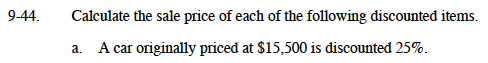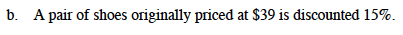### Home > MC1 > Chapter 9 > Lesson 9.1.4 > Problem9-44

9-44.Think of this as $15,500 − 2(10% of$15,500) − 5(1% of $15,500). 10% of$15,500 is $1,550. 1% of$15,500 is $155.$15,500 − 2($1,550) − 5($155)
$15,500 −$3,100 − \$775Follow the same steps as part (a).